# Using DATE function in Excel - formula examples to calculate dates

When it comes to calculating dates in Excel, DATE is the most essential function to understand. As you probably know, Excel does not keep the year, month and day for a date, nor does it explicitly store weekday information in a cell. Instead, Microsoft Excel stores dates as serial numbers and this is the main source of confusion.

Not all Excel date functions can recognize dates entered as text values, therefore it's not recommended to supply dates directly in calculations. Instead, you should use the DATE function to get a serial number representing the date, the number that Excel understands and can operate on.

## Excel DATE function

The Excel DATE function returns the serial number that represents a certain date. It has the following arguments:

DATE(year, month, day)

Where:

Year - represents the year of the date.

Month - an integer representing the month of the year, from 1 (January) to 12 (December).

Day - an integer corresponding to the day of the month, from 1 to 31.

The DATE function is available in all versions of Excel 365 - Excel 2007.

### DATE function - tips and notes

The DATE syntax looks crystal clear and straightforward on the surface. In practice, there may be some unobvious pitfalls that the below tips will help you avoid.

#### Year

Excel interprets the year argument according to the date system set up on your computer. By default, Microsoft Excel for Windows uses the 1900 system where January 1, 1900 is represented by the serial number 1. For more details, please see Excel date format.

• If the year argument is between 1900 and 9999 inclusive, Excel uses exactly the value you supplied to create a date. For example, DATE(2015, 12, 31) returns December 31, 2015.
• If the year argument is between 0 and 1899 inclusive, Excel adds the specified number to 1900. For example, DATE(100, 12, 31) returns December 31, 2000 (1900 + 100).
• If the year argument is less than 0 or greater than 9999, a DATE formula will return the #NUM! error.

Tip. To avoid confusion, always supply four-digit years. For example, if you input "01" or "1" in the year argument, your DATE formula will return the year of 1901.

#### Month

• If the month argument is greater than 12, Excel adds that number to the first month in the specified year. For example, DATE(2015, 15, 5) returns the serial number representing March 1, 2016 (January 5, 2015 plus 15 months).
• If the month argument is less than 1 (zero or negative value), Excel subtracts the magnitude of that number of months, plus 1, from the first month in the specified year. For example, DATE(2015, -5, 1) returns the serial number representing July 1, 2014 (January 1, 2015 minus 6 months).

#### Day

As well as month, the day argument can be supplied as a positive and negative number, and Excel calculates its value based on the same principles as described above.

Tip. At first sight, supplying negative values in the month or day argument of the Excel DATE function may seem absurd, but in practice it may turn out quite useful, for example in the complex formula that converts a week number to a date.

## Excel DATE formula examples

Below you will find a few examples of using DATE formulas in Excel beginning with the simplest ones.

### Example 1. DATE formula to return a serial number for a date

This is the most obvious use of the DATE function in Excel.

For example, to return a serial number corresponding to 20-May-2015, use this formula:

`=DATE(2015, 5, 20)`

Instead of specifying the values representing the year, month and day directly in a formula, you can have some or all arguments driven by of other Excel date functions. For instance, combine the YEAR and TODAY to get a serial number for the first day of the current year.

`=DATE(YEAR(TODAY()), 1, 1)`

And this formula outputs a serial number for the first day of the current month in the current year:

`=DATE(YEAR(TODAY()), MONTH(TODAY(), 1)`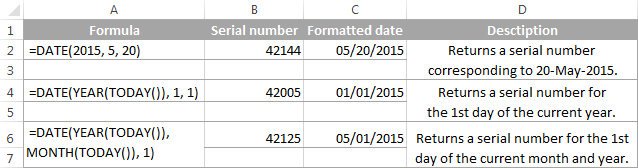Tip. To display a date rather than a serial number, apply the desired Date format to the formula cell.

### Example 2. How to return a date based on values in other cells

The DATE function is very helpful for calculating dates where the year, month, and day values are stored in other cells.

For example, to find the serial number for the date, taking the values in cells A2, A3 and A4 as the year, month and day arguments, respectively, the formula is:

`=DATE(A2, A3, A4)`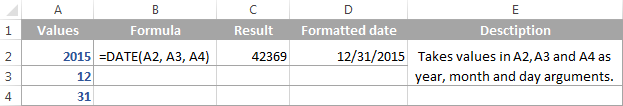### Example 3. DATE formula to convert a string or number to a date

Another scenario when the Excel DATE function proves useful is when the dates are stored in the format that Microsoft Excel does not recognize, for instance DDMMYYYY. In this case, you can use DATE in liaison with other functions to convert a date stored as a numeric string or number into a date:

`=DATE(RIGHT(A2,4), MID(A2,3,2), LEFT(A2,2))`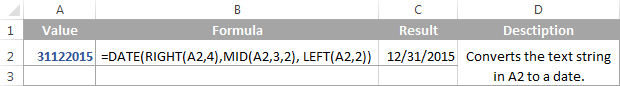### Example 4. Adding and subtracting dates in Excel

As already mentioned, Microsoft Excel stores dates as serial numbers and operates on those numbers in formulas and calculations. That is why when you want to add or subtract some days to/from a given date, you need to convert that date to a serial number first by using the Excel DATE function. For example:

• Adding days to a date:`=DATE(2015, 5, 20) + 15`The formula adds 15 days to May 20, 2015 and returns June 4, 2015.
• Subtracting days from a date:`=DATE(2015, 5, 20) - 15`The result of the above formula is May 5, 2015, which is May 20, 2015 minus 15 days.
• To subtract a date from today's date:`=TODAY()-DATE(2015,5,20)`The formula calculates how many days are between the current date and some other date that you specify.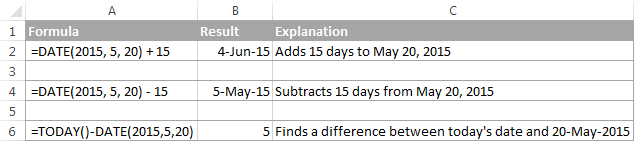If you are adding or subtracting two dates that are stored in some cells, then the formula is as simple as =A1+B1 or A1-B1, respectively.

And here are a few more examples where Excel DATE is used in combination with other functions in more complex formulas:

## Using Excel DATE formulas in conditional formatting

In case you want not only to calculate but also highlight dates in your Excel worksheets, then create conditional formatting rules based on DATE formulas.

Supposing you have a list of dates in column A and you want to shade dates that occurred earlier than 1-May-2015 in orange and those that occur after 31-May-2015 in green.

The DATE formulas you want are as follows:

Orange: `=\$A2<DATE(2015, 5, 1)` - highlights dates less than 1-May-2015

Green: `=\$A2>DATE(2015, 5, 31)` - highlights dates greater than 31-May-2015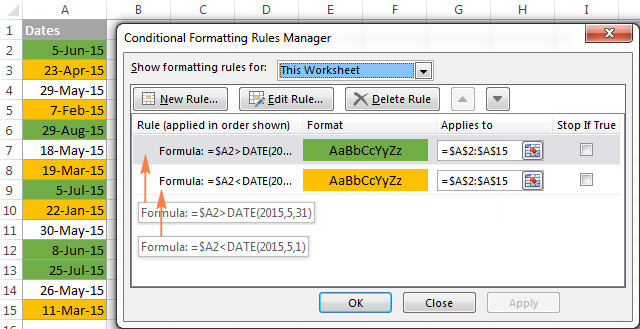For the detailed steps and more formula examples, please see How to conditionally format dates in Excel.

## How to make date formulas in Excel with Date & Time Wizard

Though DATE is the main function to work with dates in Excel, a handful of other functions are available to tackle more specific tasks. You can find the links to in-depth tutorials at the end of this article.

Meanwhile, I'd like to present you our Date & Time Wizard - a quick and easy way to calculate dates in Excel. The beauty of this tool is that outputs the results as formulas, not values. Thus you have a kind of 'two birds, one stone' opportunity - get the result faster and learn Excel date functions along the way :)

The wizard can perform the following calculations:

• Add years, months, weeks, days, hours, minutes and seconds to the specified date.
• Subtract years, months, weeks, days, hours, minutes and seconds from the specified date.
• Calculate the difference between two dates.
• Get age from the birthdate.

For example, here's how you can add 4 different units in cells B3:E3 to the date in A4. The formula in B4 is built in real-time as you change the conditions: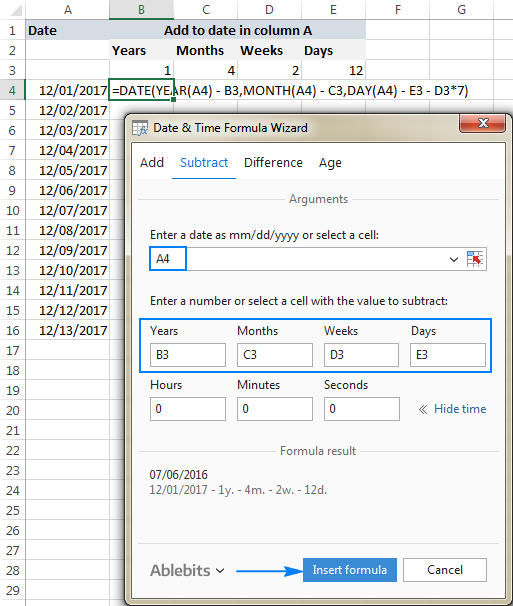If you are curious to explore other capabilities of the wizard, feel free to download an evaluation version of the Ultimate Suite below which includes this as well as 60 more time saving add-ins for Excel.

I thank you for reading and hope to see you on our blog next week!

## Excel dates tutorials:

1. Great info that you have here. Please help. I am working on my sheet of manage data,I want excel to series easily for me
kindly help me
how can i put the series in this way
for example
01/2017
02/2017
03/2017
main thing is that 01, 02, and 03 is not date or month
this is my work sheet serial number i have alot of work sheet thats way i m manage one sheet for inform me to whos my work done or not
so kindly help me how can put serial
01/2017
02/2017
03/2017
04/2017
05/2017
continues....

2. Hi
I want to count a Today date in a particular cell,Suppose my all data in sheet1 and and i prepare a formula sheet on sheet2,in that i want a count from a cell Today date only(as Like so many date are there in that i want to count how many thing dispatch on Today date),so that directly reflect to my sheet2.

3. Hi there :)

What is the best excel formula to use, to calculate the actual date based on the xx number of days + a specific date, where its counted as day 1?

The formula I'm using currently, worked correctly on some dates, but not on others, it's one day less. Could this be due to the Stat holidays within the range?

For example,
a. 2 days starting from Aug 5, 17 = Aug 6, 2017

b. 3 days starting from Aug 5, 17 = should be Aug 7, but the same formula I used for "a" returned Aug 6

c. 7 days starting from Aug 5, 17 = Aug 11, 17, but returned Aug 10.

4. how to date format change
ex: 01.01.2017

i need 01-Jan-17 format

5. Hello :)
Please let me know, How can I segregate the COLORED dates in to different columns.
Recently i did it manually but its very time taking procedure, please guide me to find an easy way.

6. Hi, I have written a macro to process dates as the X axis and the value as the Y axis. My challenges are that the length of column of dates could vary so some cells in the plotting range of the Macro could be empty (this returns default date in that cell of 01/01/1900. So my first question is how to use the VBA code so that the range is only taken with all cells containing a date and blank cells are ignored?

Next the macro will format the graph but i cant find a way to arrive at the X Axis minimum and Maximum Scale limits, if i use the "auto" function then the graph does not display until I reduce the Min and Max date number to either side of the actual date range. I thought i could use the DATEVALUE function to set the date value number as an integer and use that in the MIN and MAX graph formatting Axis code: "ActiveChart.Axes(xlCategory).MinimumScale = 42817" and "ActiveChart.Axes(xlCategory).MaximumScale = 42823". Replacing the number with the Integer for the start date and end date of my range.

7. Trying to complete task that is completed by completion date with a total of percentage.

10 task starting at B2-B11 and dates are entered to show completed how can I get B12 to show total percentage completed??

A 2-12 has names of task

any help is appreciated

8. I want to know how to remove date formula to enter other date beyond a particular date example i want to entre 10-05-17 but formula is set for 07-05-17

9. A former co-worker created a spreadsheet for our reimbursement use but the calculating/formula fields are locked down and I don't have the password. I need to recreate this spreadsheet and one of the fields/formulas has me stumped.

Column A is a date column for manual entry by users. Starting at row 9 users enter a single date (04/25/2017). Single dates can be entered up to row 54.

The formula I need is for a period of time, based on the starting and ending dates found in column A.

This is what the locked field looks like with no data in column A: "From 1/0/00 to 1/0/00"

This is what the locked field looks like with data in column A, row 9 (1st row of data) & row 12 (last row with data): "From 04/24/2017 to 04/25/2017".

My TEXT formula is not correct
(=TEXT(A9,"mm/dd/yy")&" - "&TEXT(A54,"mm/dd/yy")

The result is this:

"04/20/2017 - 01/00/00" (even though I have data/dates in rows 10-17)

I'm not sure how well I have explained myself but any help is greatly appreciated. Thanks!

10. hi, pls i will like you to help me with the steps on how to convert text into numerical values Using Excel 2010.

11. Hello,

I am attempting to calculate the inventory value based on the inventory quantity (in column B) and today's unit price (columns C through I represent the days of the week and have unit prices below each day for each item; I have a new sheet for each week). I want the inventory value to display in column K following each type of item in my inventory (column A for each item).

• Hello, Spinky,
If the starting date is in A1, then you place the following formula in A2:
=DATE(YEAR(A2) + 5, MONTH(A2), DAY(A2))
Check the link to see DATE function in more details.

13. Hello please help. What is the calculation for this. I want to get the average and/or sum.

ColumnA: 15 days, 1 hours
ColumnB: 15 days, 6 hours

Thank you

14. Hi,

I got a calculation to do in excel and I am completely stuck. I need to calculate if a staff is eligible for local leave or not. The staff is entitled local leave after 1 year (365days). So, if I have a date of entry 9/04/2017 in the next cell it should say YES if not 'NE' Not Eligible.

Can someone help me on this please?

Br,
John

• Hello, John,
assuming that the dates are in A row, you need to calculate the following: date of hiring (A1) – date of entry (A2) = result (A3).
Try the next formula in A3:
=IF((A2-A1)>=365, "YES", "NE")

15. hi, can you help me with some date formula, i wanted to put formula in cell A1 where when you write anything on cell B1, the cell A1 will automatically generates a date for that day.... and on the next day, when you write on cell B2 the the cell A2 will automatically generate a date on the same day but will not affect the cell A1... meaning if today is January 1, 1900 and write anything on cell B1, cell A1 will be dated January 1, 1900 and when i write tomorrow on cell B2, cell A2 will be dated January 2, 1900 but cell A1 will still be January 1, 1900.... hope you can help me.

16. Hello,
I have a table with formulas containing dates in them. Is there a way that these cell formulas automatically update with new results every time I put in new weekly dates in a separate column?
(Example: Columns S6 till S12 - Sunday, 3/26 till Saturday 4/01)

17. I figured out how to calculate dates based off of a start date, however, when that initial cell is blank, it fills in random dates that I don't want to be visible. Is there a formula that I can embed into the cell so that they stay blank till the initial cell is filled?

• Hi, Johanna,
if the initial cell is A1, try the following:
=IF(ISBLANK(A1),"",FORMULA)
Note, that instead of the word FORMULA you put that formula of yours to calculate the date.

18. Hi
i'm looking for a formula to get service years on a specific date. For example, joining date 01/01/2015 in cell A1, what is the formula to get years on 31/12/2016 ?

19. Hello Everyone

I've been looking everywhere for help to my edate error but cant find anything that works. Hope someone here can help.

I am trying to use edate to subtract 12 months from a given date to use in a look up calculation for Fiscal YTD results. Everything works fine until February 2017 rolled around and the results give me February 28, 2016 not February 29, 2016. Which causes a #N/A error.

=IF(B8=EDATE(Q3,-12),B10,SUM(B10:INDEX(B10:Y10,1,MATCH(EDATE(Q3,-12),B8:Y8,0))))

Is the formula I am using which works fine for every other month.

Q3 refers to the current month end date: in this case: 2/28/2017

Row 8 has dates in subsequent cells as follows:
7/31/15 8/31/15 9/30/15 10/31/15 11/30/15 12/31/15 1/31/16 2/29/16 3/30/16 etc all the way up to my 2/28/17 month.

the formula returns: 2/28/16 not the 2/29/16 I need to get my prior fiscal year to date total.

In other words my formula returns the date as 42428 not 42429. Which the causes the formula to error out since there is no 42428 in the date cells.

Thanks in advance for the help.

20. HELLO DEAR
I WANT SHOWN DATE IN EXECL IN BELOW PATTERN
12/05/2016
I WRITE 120516 AND ITS SHOW AS 12/05/2016

21. what formula can I use to calculate the minimum \$ value in a colum from todays date forward for every time I open my spread sheet.

22. You guys are great! looks like you've helped a lot of people. Here is another one for you.

Im trying to format columns based on an start date and an end date. I've been able to insert a start date and get the following months to populate by using =EOMONTH(\$A\$7,C\$1) A7 being start date and row one being by number of months following. As it is now i have add or subtract columns manually to get to the correct end date. Then if i change the beginning date all the cells formatted below are in the wrong months.

If you can it'd be great to get some help thanks.

23. I just found your blog and am amazed at the detail and depth of your responses! Quite impressive! I want to create a macro for entering payment data into certain rows of column M (where each row is one day) based on options from a drop down menu (accessed when any cell in column M is right clicked) and based on the date in column A. The drop down menu options are: amount, start date, frequency (1 week, 2 week, 4 week, monthly), and number of payments (max 500). If the entry falls on a weekend, it should be moved back to fall on the Friday before. The first entry will be on the same row as the row of the date in column A and the remaining entries similarly. I know this is fairly complex and thank you so much for your help!

24. Hi, i am property broker and i want to yellow colour in after 330 days and Red colour after 360 days formula reminder. plz help.

25. I have a cell downloaded from a web report that contains 13/02/2017 15:30:00 all in one cell.
How can I extract the date, and then the time into two separate cells further along the page?

26. Hi, I am trying to use this formula to calculate date difference but I also want the formula to return 0 or do nothing for lines without dates.
Formula used is =DATEDIF(Q5, TODAY(), "d")/7
The problem is once I drag down it also fills the lines with blank dates as 6111 which is incorrect but lines with dates are correct.

27. How do you calculate the number of days from start to end and adding 1 to the results?
Start : 6/4/2018
End : 6/6/2018

28. Enter a formula in cell G5 that calculates the difference between the attendance totals for 2018 and 2017. Copy the formula to the range G6:G11.

29. Hello,

I need a date formula for example:

The two dates are set at 09/01/2016 -10/01/2016 calculating the daily rate at £44.02 but I need it to acknowledge the two dates as two days not one and return the daily rate as £88.04

30. Hi, I am trying to create a formula that will return a result if the current month is December or if the date range is between Dec 1 - Dec 31 (not specific to year), otherwise return 0.

I have this so far... but it is dependent on the year being there and being updated every year. How can I make it so that it is any December ?

=IF(AND(TODAY()>=DATE(2017,12,1),TODAY()<=DATE(2017,12,31)),'DR Schedule B'!F31,"0")

• I have found one that works!!! Thanks

31. I want to enter a birthday, then calculate the age for a specific day.

Example: Child is born 10/7/10. How old will he/she be on 10/19/17?

32. Dear,

I need a easy excel formula see below example

to 24-11-16 from present 4-1-17 = - due day

Also need = date-month -year

Best Regards

Russel

33. i have a problem in excel that is when i am entering 1-1, 1-2, 1-3 ,....... 1-11,1-12 it is converting into 01-jan, 01-feb,...... 01-nov, 01-dec . How to convert them into numbers when i am entering 1-13 it stay in 1-13

plsss give a detailed solution for my problem

• To get the number as you required is to add ' before entering number.
put '1-1, '1-2 and if you drag you will see

34. Hi,
Many thanks for suggesting with the right formulas
Well i need a formula that particular date data should change if i change the date at the top
Like In B column dates will come and in the remaining columns some other data. in other sheet if i want some particular "date" data as front page columns here rows and the data should match with the date
Plssssss help me

35. Hi there,
I am trying to input a formula. I have a worksheet with 2 tabs.

Tab one is the worksheet I input dates and details into.

Tab 2 has pricing or rates for each day of the year.

I want my worksheet on tab one to recognise that when I enter a date it populates that rate for the particular day from tab 2

Can someone assist?

36. I am working on completing an excel file that acts like a library book checkout/return listing. I was looking to find a way to have Excel see a date in one cell and automatically give a result of a date 60 days later within a different cell. I found the formula below on your site but was wondering how I could have the date automatically pop up when I input a checkout date in a different cell. Sort of like a conditional command. For example: If I place 1/27/2017 in A2, I'd like 3/28/2017 to automatically show up in cell D2. How do I accomplish this command?

=DATE(2015, 5, 20) + 15

The formula adds 15 days to May 20, 2015 and returns June 4, 2015.

37. I am trying to have a cell show a date 30 days less than the date in another cell. So cell A1 has 03/31/17. I want cell A2 to calculate A1 minus 30 days. Please help!

• Never mind. When I actually tried in Excel, super easy. I am trying to find an IF formula that I can use in Smartsheet to get this done. The simple =A1-30 that works in Excel is not working in Smartsheet.

38. Hello, I am trying to subtract one date from today's date. For example

A1 = (Review Date) which has a date of 01/27/2017
Todays Date is 1/25/2017

But my formula returns -2. It should return 2 without the negative.
Here is my formula

=TODAY()-[@[Review Date]]

39. 01/01/2017 14:03:12
how to single Cell IN EXCEL pls help me

40. Hello!

I love your tutorials! I do have a question though.
I have a documentation sheet that has "Move In" dates and "Move Out" dates for renters in their own separate columns. I also have a column that counts the days from the date that they first move in. For example Move In Date is 12/25/2016 so the current number of days in house according to today's date would equal 25. However the formula I am using currently continues counting from the move in date to the current date (today). How would I get my counter to stop counting based on the move out date and retain the counting ability?

41. Hello! Could you help me with a formula to add (sum) two periods. For example if I wish to add 01 year, 06 months, 12 days with 02 years, 07 months, 26 days how can I do it? With what formula? The correct answer would be 04 years 02 months and 08 days. But what formula in Excel would give me that answer. Thank you.

42. my dog show is on 28/1/17
i want to calculate age from 4 months to 6 months, 7 months to 12 months, 13 months to 18 months, 19 months to 36 months from cut off date which is 28/1/17

43. Hello,

I have a start date and I have it so it tells me how many days open, but how would I get that to stop when I put in a date completed?

Thanks

44. I would like to calculate 18 months from each of the dates for an entire column?

45. hi ,
i would like to make a formula that automatic increase the value every year in April or if i change the cell to month April other cell automatic the value with the percentage increase

46. Hi,

How to find out the due date for one who completes the probation period i.e. 6 months. The due date should be in DD/MM/YY. Please help.

47. AMAZING SITE! ALL ANSWERS ANSWERED SPECIFICALLY.i would like to create a calendar with events, but i would also like the events to be automatically written out in the calendar.
is there any resources on your site that helps?
thanks.

48. Hello, I have used this site many times looking for solutions to my Excel problems. I am trying to figure out a way to add to dates in different columns. I am tracking things weekly beginning on Monday and ending on Sunday. Short of manually adjusting every cell, is there a formula to fix?
Example:
C1 is 1/1-1/8
D1 is 1/9-1/15
E1 is 1/16-1/22
F1 is 1/23-1/29
G1 is 1/30-2/5
etc. every week to 12/31

49. Hi,
how may I convert a given sample/answer (example: 3d 10h 35min) in a certain cell into a total minutes only?

Thank you.

50. Hi,

I have a date in cell B2. I also have a a table from A5 to B8 (Column A are date values and Column B are price values).

I need for Excel to use the date in B2 to search for the latest date in Column A before the date in B2, and throw me back the price from Column B.

For example:

B2=01/05/2017

A5=01/01/2017
A6=01/01/2017
A7=01/03/2017
A8=01/15/2017

B5=\$3.00
B6=\$3.00
B7=\$3.50
B8=\$3.70

I need the formula to give me the \$3.50 price.

Can you please help me? I know it seems simple, but have been having a hard time with it.

Sincere regards